# 2D Array in Python

Challenge Inside! : Find out where you stand! Try quiz, solve problems & win rewards!
Learn via video coursePython Course for Beginners With Certification: Mastering the Essentials
By Rahul JanghuFree
4.90Enrolled: 1000Python Course for Beginners With Certification: Mastering the Essentials
Rahul JanghuFree
4.90
Enrolled: 1000

## Overview

A 2D array in Python is a nested data structure, meaning it is a set of arrays inside another array. The 2D array is mostly used to represent data in a tabular or two-dimensional format.

## Introduction to 2D Array in Python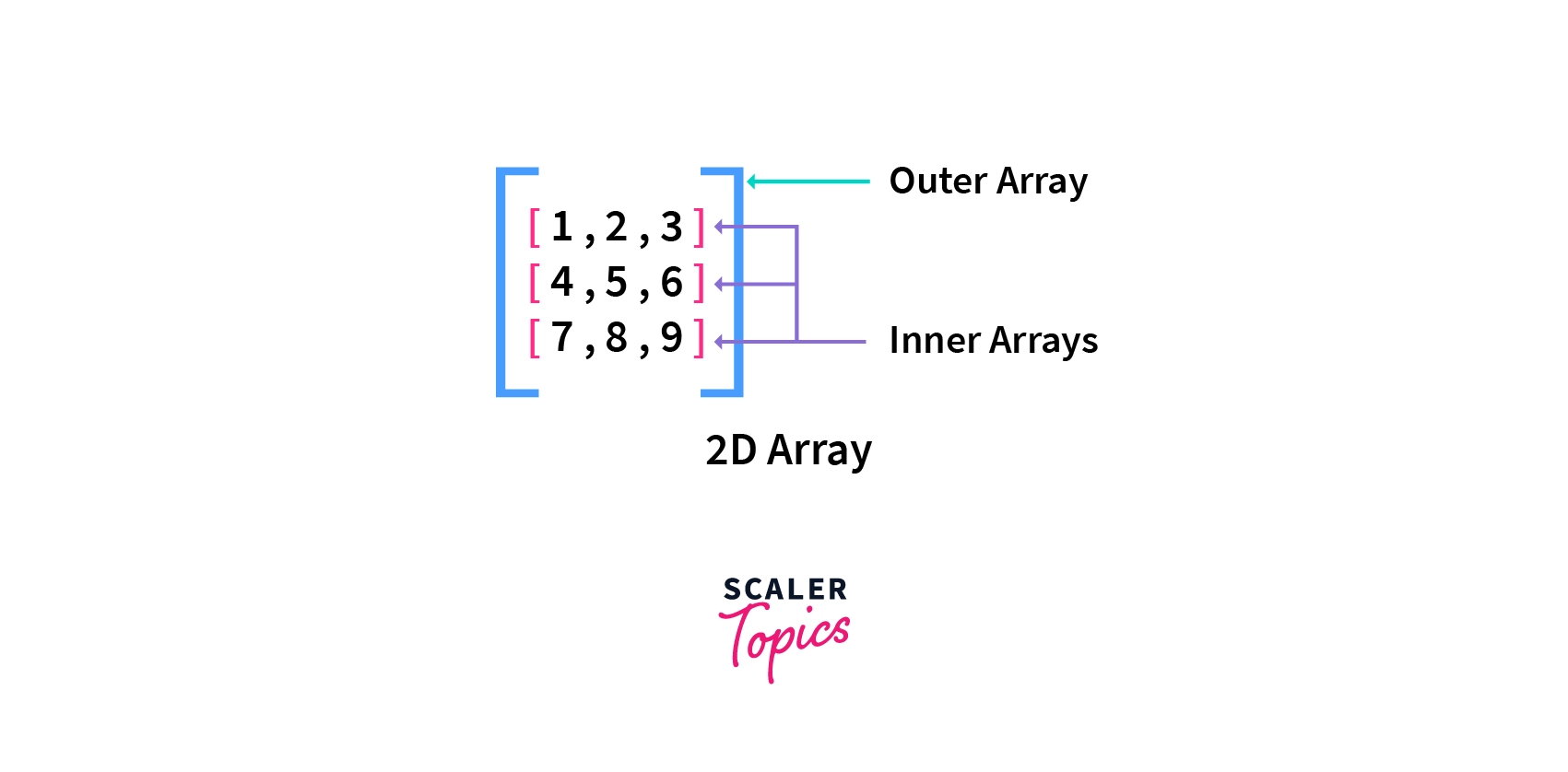A 2D array in python is a two-dimensional data structure used for storing data generally in a tabular format. For example, if you want to store a matrix and do some computations, how would you do it in Python? Well, the answer is the use of 2D arrays in Python. Read along to know more.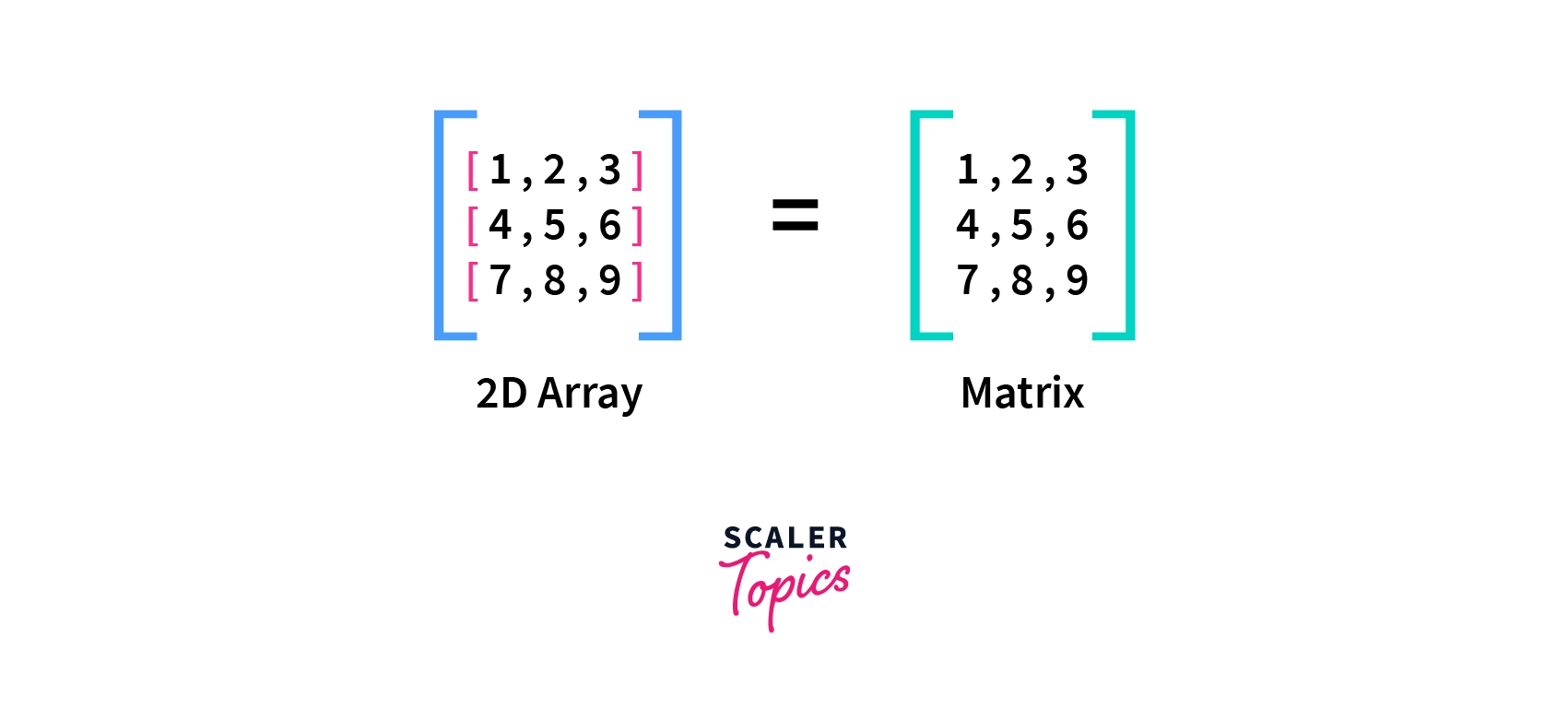## What is 2D Array in Python & Its Uses

A 2D array in python is a two-dimensional data structure stored linearly in the memory. It means that it has two dimensions, the rows, and the columns, and thus it also represents a matrix. By linear data structure, we mean that the elements are linearly placed in memory, and each element is connected to its previous and next elements.

We visualize a 2D array in a tabular format, but actually, elements are stored linearly in memory. Elements of a 2D array are contiguously placed in the memory. It means that if one element is present at the current memory address, the next element will be placed at the next memory address. This type of storage makes arrays randomly accessible. It means that we can access any element of the array independently.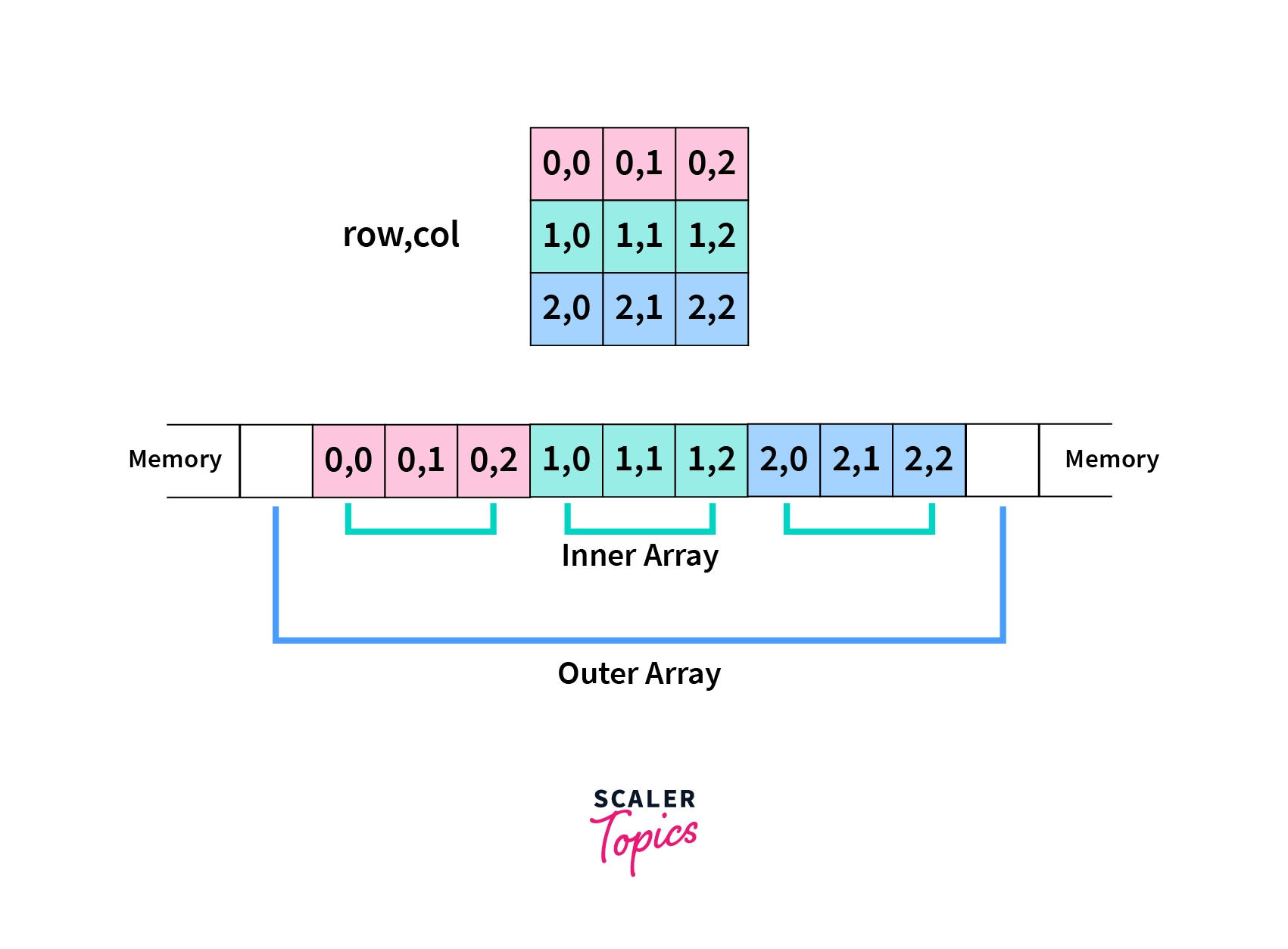In a 2D array, multiple arrays are inserted as elements in an outer array. Each element in a 2D array is represented by two indices, the row and the column. 2D arrays in python are zero-indexed, which means counting indices start from zero rather than one; thus, zero is the first index in an array in python.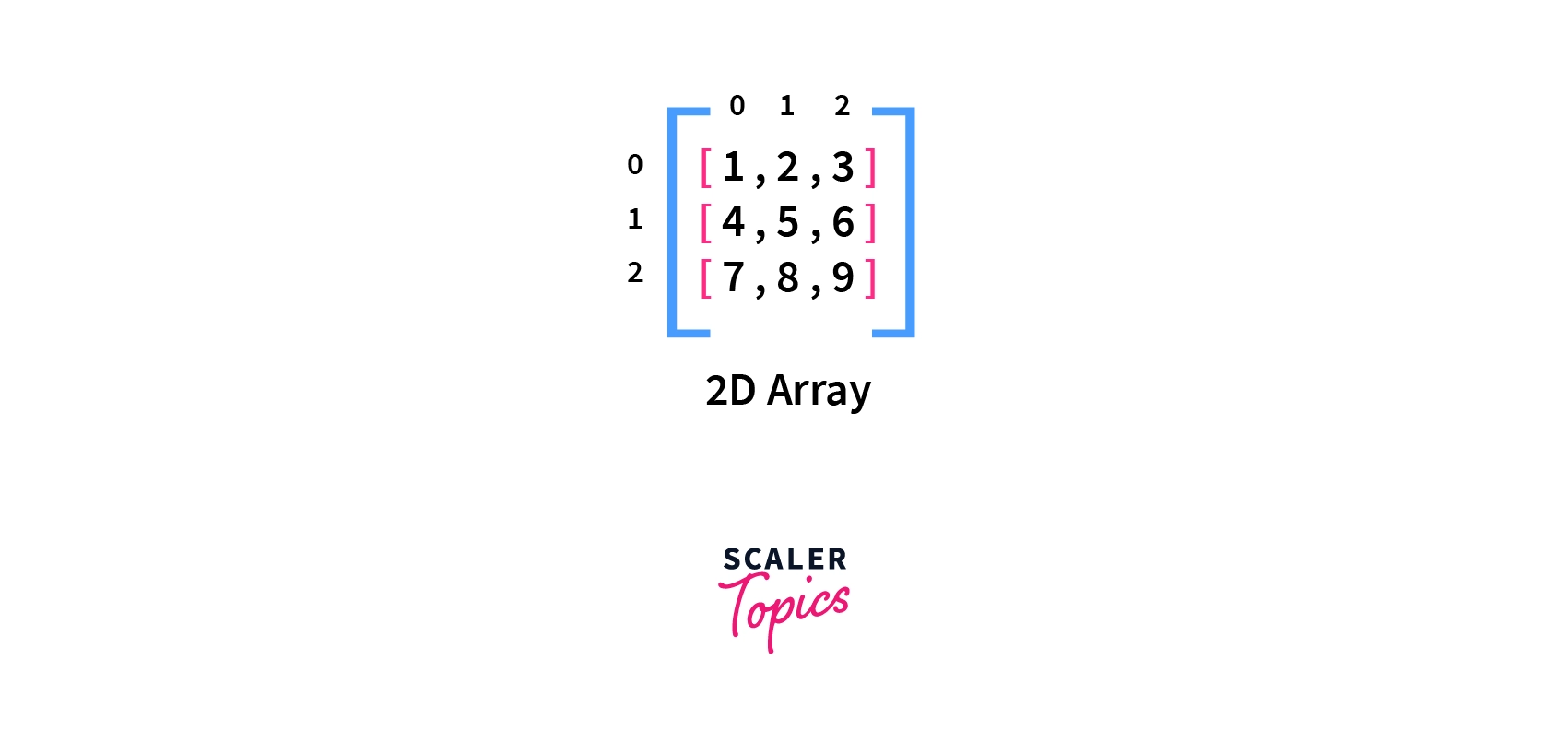2D arrays are used wherever a matrix needs to be represented. We can create a 2D array with n rows and m columns representing an mxn matrix.

2D arrays are also used to store data in a tabular format. 2D arrays are also used to represent a grid while working with graphics, as the screen is also represented as a grid of pixels.

## Syntax of Python 2D Array

We can create a 2D array in python as below:

array_name=[n_rows][n_columns]

Where array_name is the array's name, n_rows is the number of rows in the array, and n_columns is the number of columns in the array.

This syntax only creates the array, which means that memory space is reserved for this array, but we will add the values later.

There is another syntax for creating a 2D array where initialization (creation of array and addition of elements) is done with a single line of code. This syntax is as follows:

Where array_name is the array's name, r1c1, r1c1 etc., are elements of the array. Here r1c1 means that it is the element of the first column of the first row. A 2D array is an array of arrays.

## Accessing Values in Python 2D Array

We can directly access values or elements of a 2D array in Python. It can be done by using the row and column indices of the element to be accessed. It has the following syntax:

Where array_name is the array's name, row_ind is the row index of the element, and col_ind is the column index of the element.

If we specify only one index while accessing an array, this index is treated as the row index, and the whole row is returned. It has the syntax:

Where array_name is the array's name, row_ind is the row index to be accessed.

If an index exceeds the size, if the array is provided to this function, it returns the index out of range error. Such indices are known as out of bounds. It is further clarified by the examples below.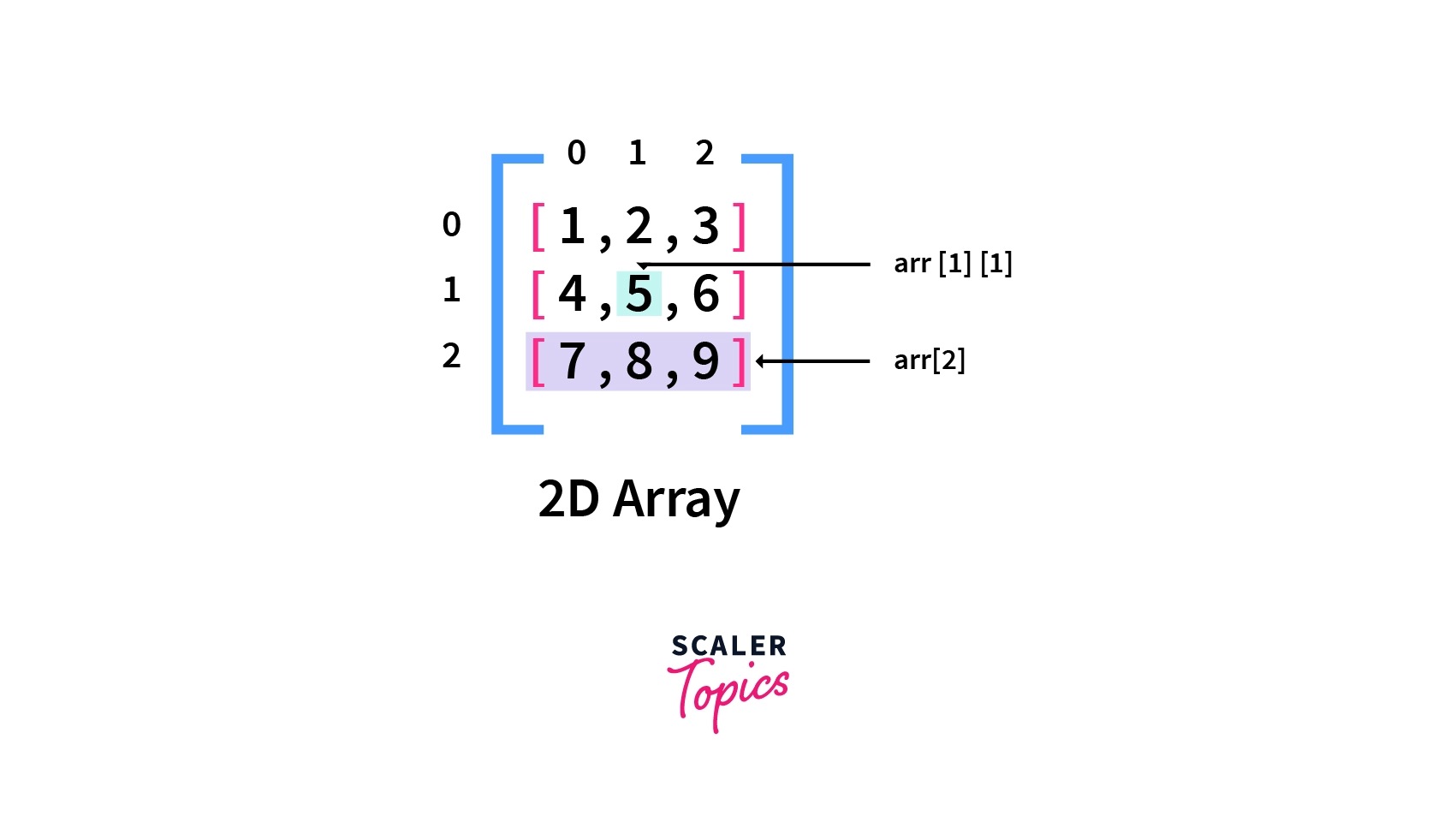### Examples of Accessing

Let's see some examples of how elements are accessed in a 2D array in python.

### 1. Accessing Single Element

In this example, we first initialize an array named arr with the given values, then access a single element of the arr array present at the 3rd column in the second row. As arrays are 0-indexed, we will use the values 1 and 2.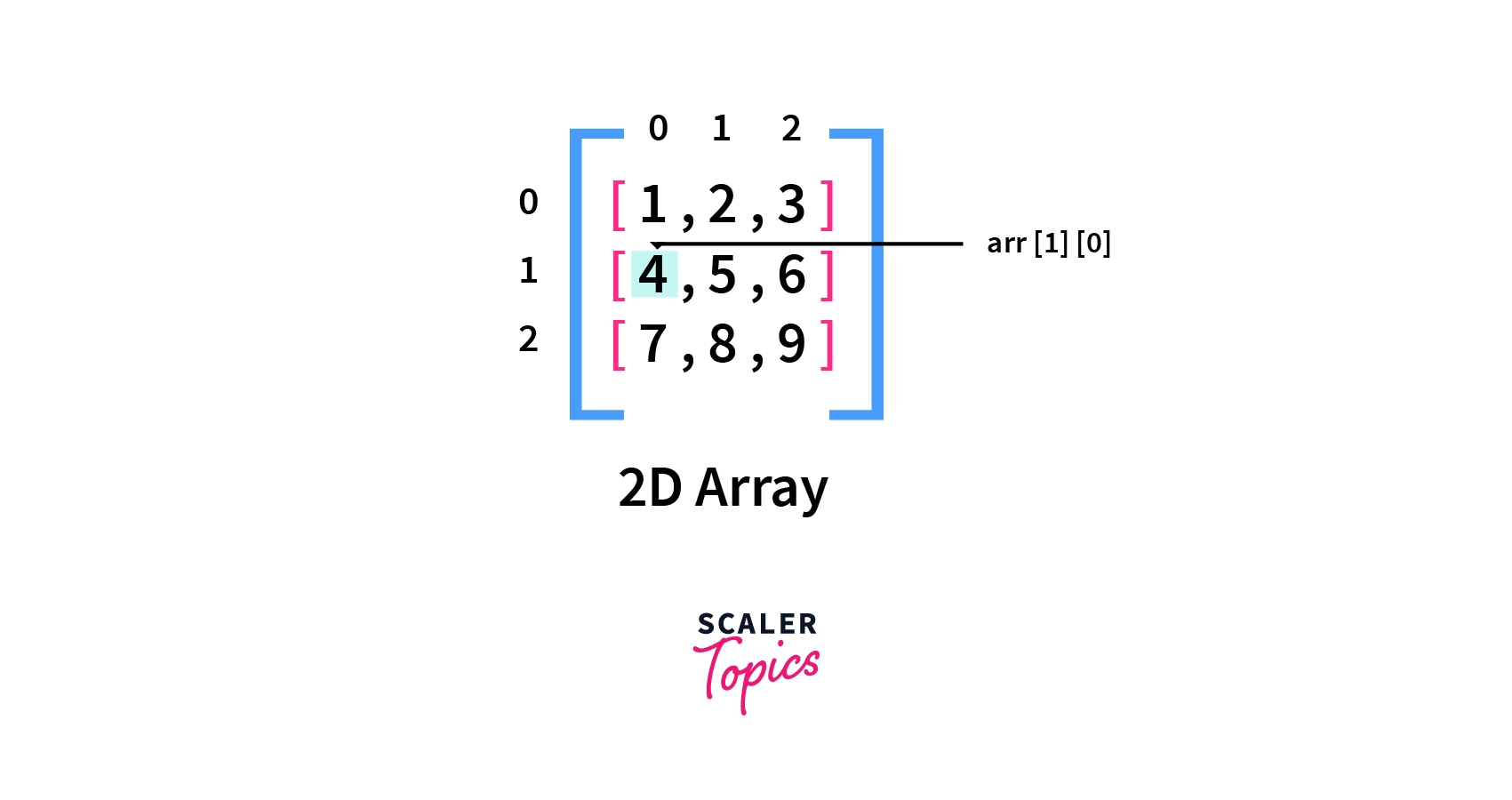Code:

Output:

### 2. Accessing an Internal Array

In this example, we only pass the row index, i.e., 2, to access the 2D array arr. At that location, another array is present, and thus that array is returned to us.

Code:

Output:

## Traversing Values in Python 2D Array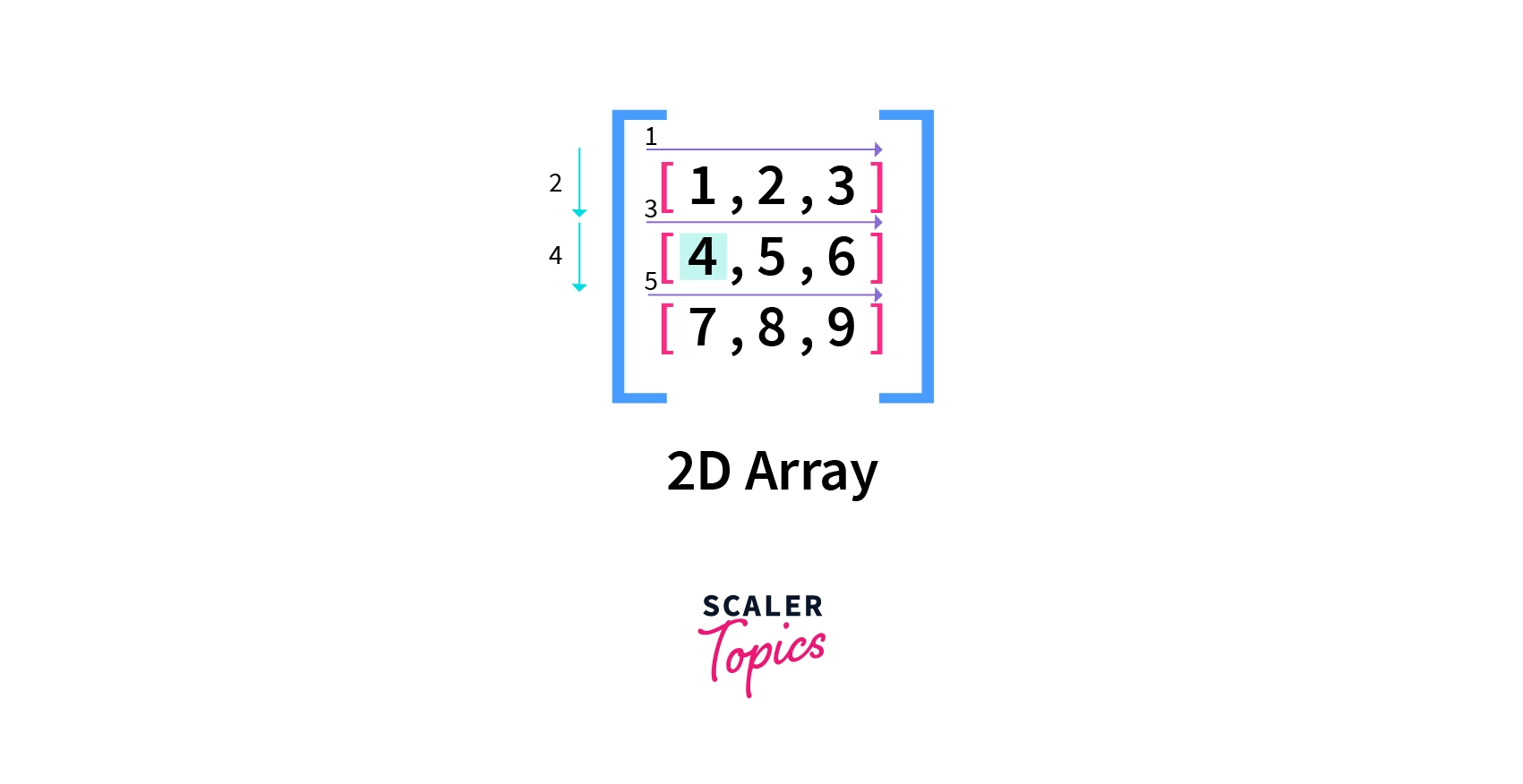Traversing means sequentially accessing each value of a structure. Traversing in a 2D array in python can be done by using a for a loop. We can iterate through the outer array first, and then at each element of the outer array, we have another array, our internal array containing the elements. So for each inner array, we run a loop to traverse its elements.

As you can see in the above image, the arrows are marked in sequence. The first row is traversed horizontally, then we come down to the second row, traverse it, and finally come down to the last row, traversed.

### Example of Traversing

Let's see some examples of how elements are traversed in a 2D array in python.

In this example, we traverse the array named arr by using two for loops. The first for loop iterates the outer array, returning the internal arrays and the second for loop traverses each of the inner loops, returning the elements.

Code:

Output:

## Inserting Values in Python 2D Array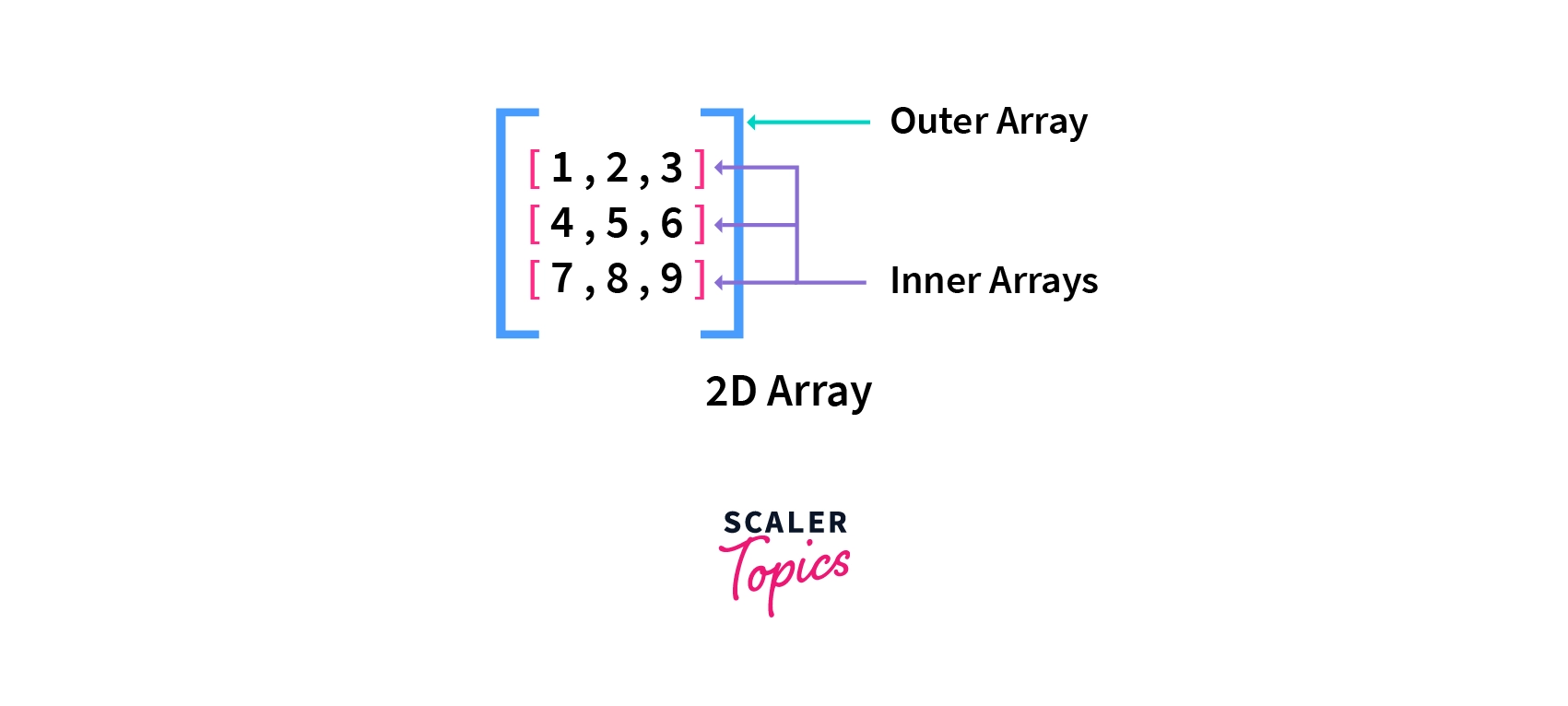Inserting a value in an array means adding a new element at a given index in the array and then shifting the remaining elements accordingly. Inserting a value would cause the values after to be shifted forward.

We can add values in a python 2D array in two ways:-

### 1. Adding a New Element in the Outer Array.

We must recall that a 2D array is an array of arrays in python. Therefore we can insert a new array or element in the outer array. This can be done by using the .insert() method of the array module in python; thus, we need to import the array module. The insert method has the following syntax:

Where arr1 is the array in which the element is inserted, ind is the index at which the element is inserted, and arr_insert is the array or element to be inserted.

### 2. Adding a New Element in the Inner Array.

As we have seen in python, a 2D array is an array of arrays so that we can add an element in the inner array. This can be done using the .insert() method of the array module in python; thus, we need to import the array module. In this case, instead of adding a value to the outer array, we add it to the inner array and thus specify the index of the array element where inside which the element will be added. Here we use the syntax as:

Where arr1 is the outer array in which the element is inserted, r is the index of the required array in the outer array, ind is the index at which the element is inserted in the inner array, and arr_insert is the array or element to be inserted.

### Examples of Inserting Values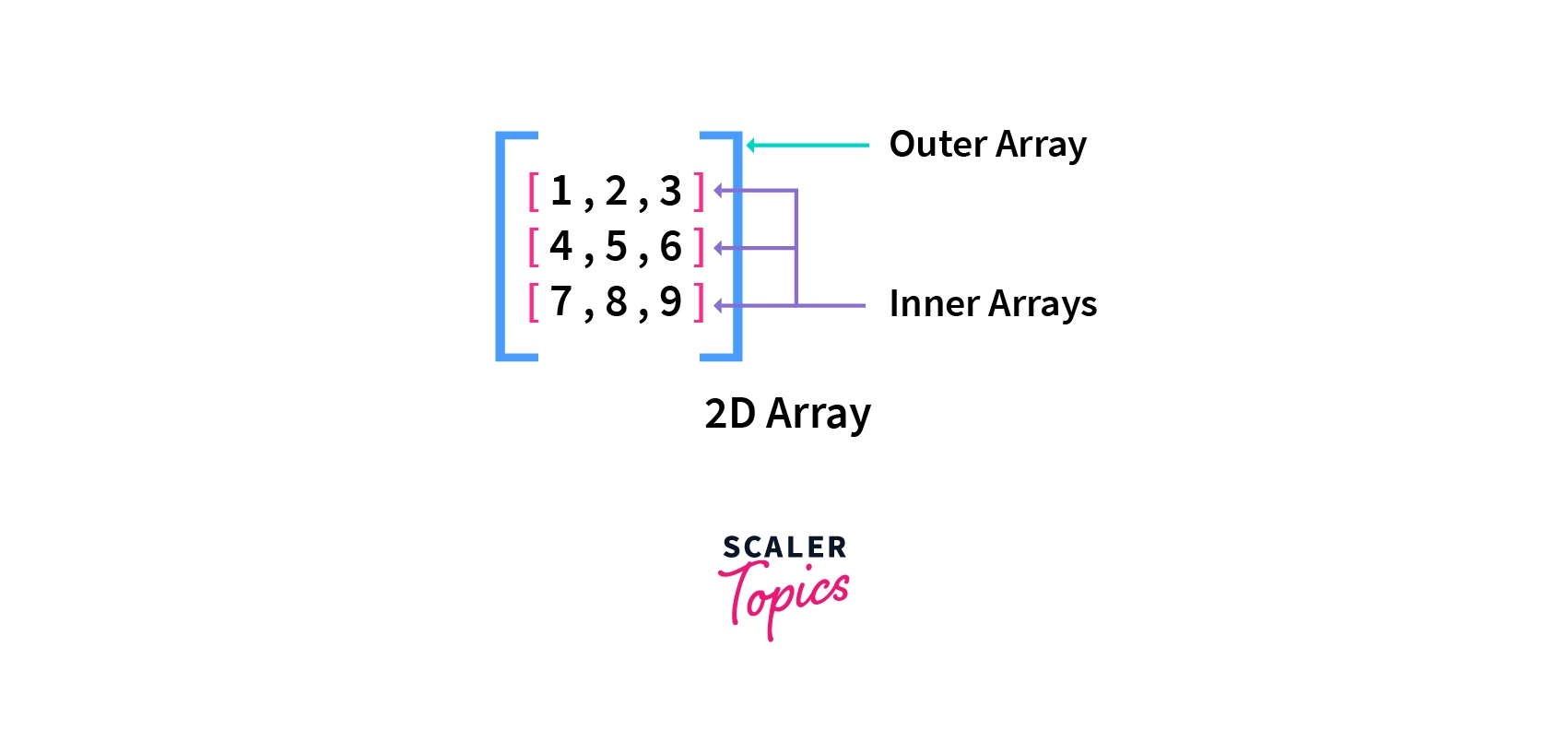Let's see some examples of how elements are inserted in a 2D array in python-

### 1. Inserting an Array in a 2D Array.

In this example, we are using the array module of python. Here we are inserting an array [11,12,13] at index 2 in our already existing array arr. It causes the values after index 2 to shift ahead. If an element is inserted at an index that is out of bounds(greater than the last index of the array or smaller than the first index), we do not get an error, but the element is inserted at that index.

Code:

Output:

### 2. Inserting an Element in Inner Array.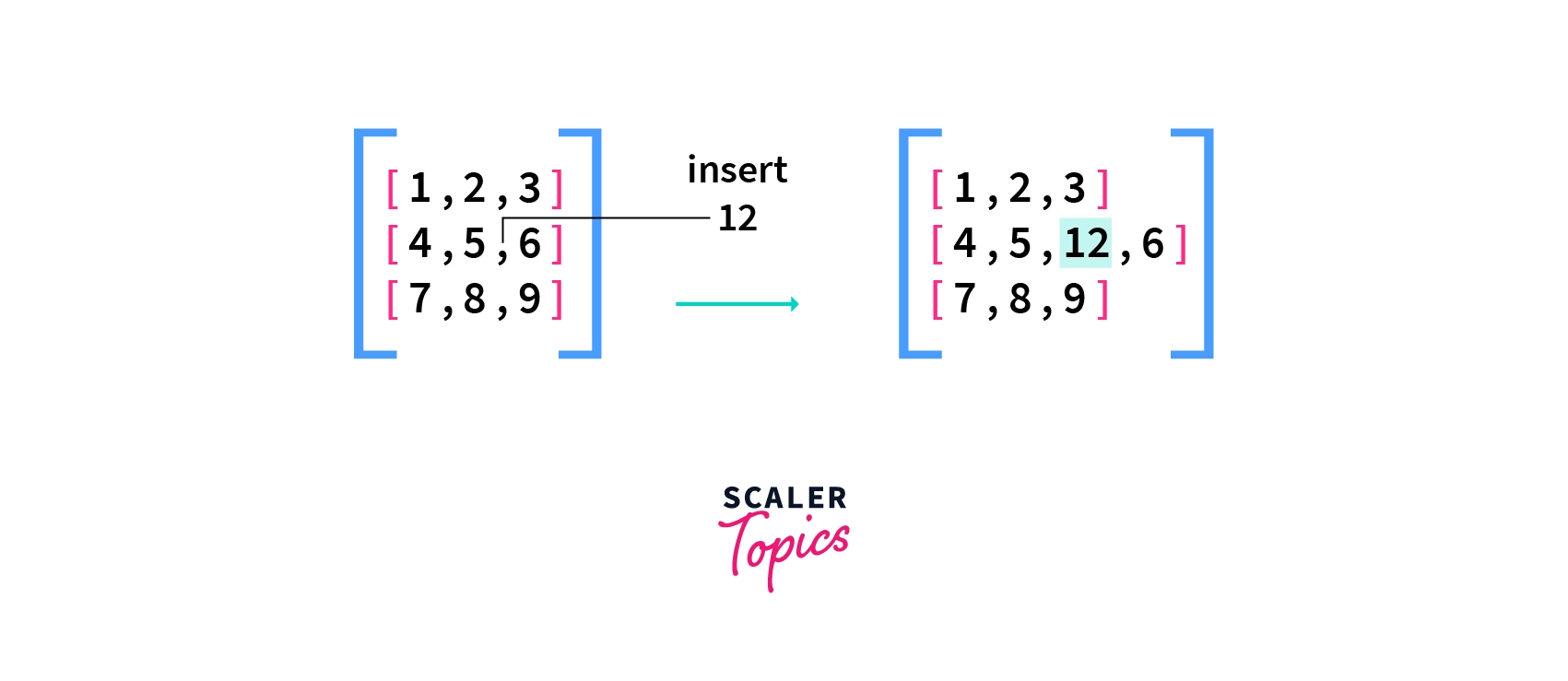In this example, we insert an element 12 at index 2 in our inner array having index 1. For doing this, we are applying the insert function on arr, thus using it as our primary array for insertion. If an element is inserted at an index that is out of bounds(greater than the last index of the array or smaller than the first index), we do not get an error, but the element is inserted at that index.

Code:

Output:

## Updating Values in Python 2D Array

Updating in a 2D array means changing an existing element's value. Updating in python 2D array can be done in two ways -

### 1. Updating a Single Element

We can update a single element in a 2D array by specifying its row and column index and assigning that array position a new value. Assigning a new value overwrites the old value, thus updating it. If we try to update a value at an index that is out of bounds(greater than the last index of an array or smaller than the first index), we get the error list index out of range.

It has the following syntax-

Where arr_name is the name of the 2D array in which the updation is done, r is the row index of the element to be updated, c is the column index of the element to be updated, and new_element is the new value to be updated to

### 2. Updating an Inner Array

We can also update an array inside our outer array. This can be done by specifying its index in the outer array and then assigning that array position a new array. The new array overwrites the old array. If we try to update a value at an index out of bounds(greater than the last index of the array or smaller than the first index), we get the error list index out of range.It has the following syntax:

Where arr_name is the name of the 2D array in which the updation is to be done, ind is the index of the element to be updated, and new_array is the new array to be updated.

### 1. Updating a Single Element

In this example, we have updated the value in the 2nd column in the 1st row to 16. It causes the old value to be overwritten with the new value.

Code:

Output:

### 2. Updating an Inner Array

In this example, we are updating the array at index 1 in the outer array with a new array named new_arr. This overwrites our old inner array with a new array, thus updating it.

Code:

Output:

## Deleting Values in Python 2D Array

Deleting values in python means removing a particular element from the array. Deleting can be done by using the array module of python. This causes the elements after it to be shifted back. Deleting in a 2D array can be done in 2 ways:

### 1. Deleting a Single Element

We can delete a single element in a 2D array by specifying its row and column index and applying the del() method on it; thus, we need to import the array module. If we try to delete a value at an index that is out of bounds(greater than the last index of the array or smaller than the first index), we get the error list index out of range.

It has the following syntax-

Where arr_name is the name of the 2D array in which the deletion is done, r and c is the row and column index of the element to be deleted.

### 2. Deleting an Inner Array

We can also delete a complete array from the outer array. This can be done by applying the del method on an array element of the outer array by specifying only the inner array's index; thus, we need to import the array module. If we try to delete a value at an index out of bounds(greater than the last index of the array or smaller than the first index), we get the error list index out of range.It has the following syntax:

Where arr_name is the name of the 2D array in which the deletion is to be done, and ind is the index of the element to be deleted.

### 1. Deleting a Single Element

In this example, we have deleted the value in the 2nd column in the 1st row. It causes the values after it to be shifted one index back.

Code:

Output:

### 2. Deleting an Inner Array

In this example, we delete the array at index 1 in the outer array. It causes the internal arrays after it to be shifted one index back.

Code:

Output:

## Inner Working of Python 2D Array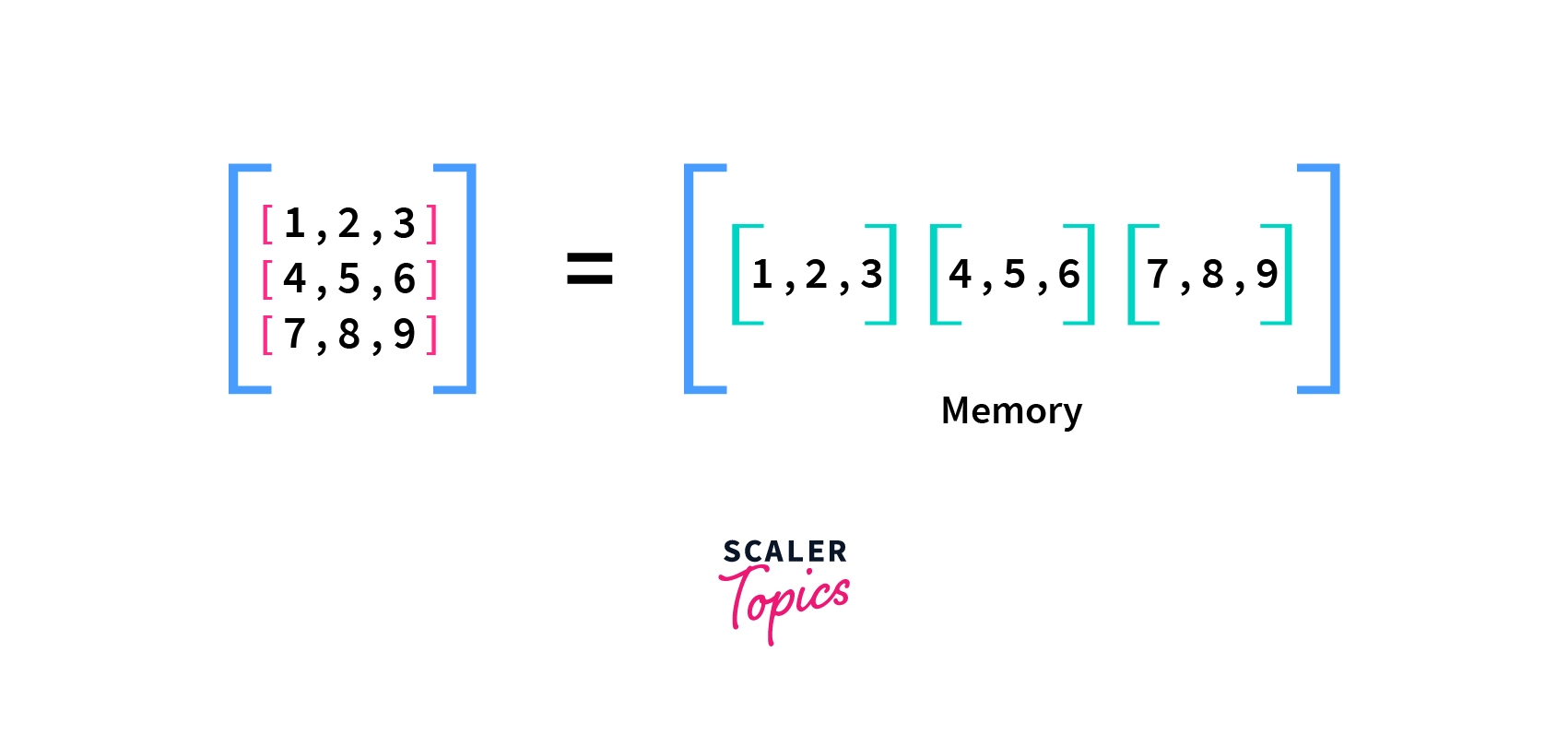We have seen above that Python 2D arrays are stored linearly in memory. The above image shows that the array is stored linearly in memory. The elements of the first row of the 2D array are stored contiguously in memory, followed by the elements of the second row and so on. The next question is how an element's memory address is calculated.

We always know the memory address of the first element of the first row. Finding the memory address of elements of the first row is pretty simple as it is equal to the memory address of the first row's first element plus the element's index multiplied by size of element.

The equation becomes a little complicated for further rows as we need to consider all the previous rows. So the address of an element becomes equal to the sum of the memory address of the first element of the first row, plus the number of elements in each row multiplied by the number of previous rows plus the column index of the current index, multiplied by the size of one element in the array.

The following formula would make the concept clearer-

Address(arr[i][j]) = B + (i * C + j) * size

Where i and j is the row and column index of the element, B is the base address of the first element of the first row, C is the number of elements in each row, size is the storage size of one element in the array, and arr[i][j] is the memory address of the element at the $jth$ column in the $ith$ row.

## Conclusion

• An array is a collection of linear data structures containing elements of the same data type in contiguous memory space.
• It is like a container that holds a certain number of elements with the same data type.
• An array's index starts at 0; therefore, the programmer can easily obtain the position of each element and perform various operations on the array.
• A 2D array is an array of arrays that can be represented in matrix form, like rows and columns. In this array, the position of data elements is defined with two indices instead of a single index.
• In Python, we can access two-dimensional array elements using two indices. The first index refers to the indexing of the row and the second index refers to the column.